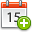# Descriptive Complexity of Graph Classes via Labeling Schemes

26th May 2021, 14:00Maurice Chandoo
FernUniversität in Hagen

## Abstract

Labeling schemes are space-efficient data structures that can be used to represent graphs from certain classes such as planar graphs or interval graphs. The idea is to assign short labels to each vertex of a graph such that the adjacency of two vertices can be computed from their labels. The algorithm which computes this is called label decoding algorithm. The time complexity of this algorithm should be low since it corresponds to the time to query an edge in the graph.

If we restrict the complexity of the label decoding algorithm, what graph classes still admit a labeling scheme? Surprisingly, even if the label decoder must be computable by a family of boolean circuits of constant-depth and polynomial size (AC0), every natural (=hereditary) graph class which is known to have a labeling scheme still can be found in this class. Alternatively, the label decoders of many labeling schemes can be described by FO formulas. Therefore FOL provides a different and in a sense more restrictive framework from classical complexity classes that can be used to analyze the descriptive complexity of graph classes.

In this talk I will give a general introduction to labeling schemes, different complexity classes of graph classes defined in terms of labeling schemes and graph parameters such as tree-width, how they relate and some basic properties. Moreover, a reduction notion among graph classes is described that makes it possible to apply the concept of completeness in this context. Finally, some complexity- and graph-theoretic research questions in this direction are presented.

The estimated talk time is 30min.